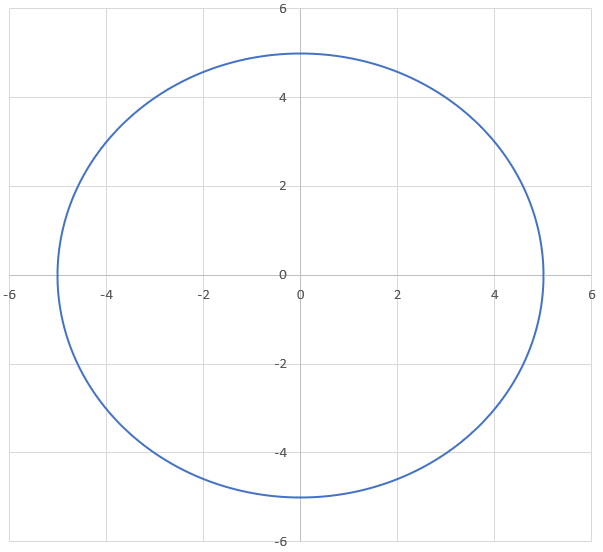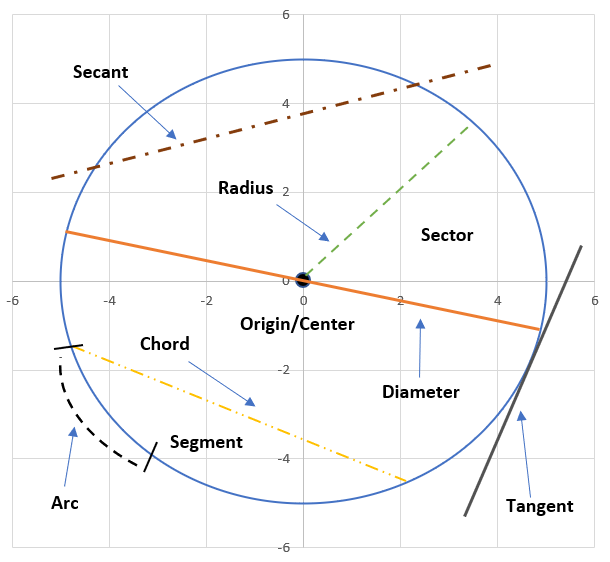# Properties of Circles

A Circle is a 2-D figure that is formed by a set of points that are at a fixed and equal distance from a fixed point in the plane, the center of the circle, commonly referred to as the Origin.## Characteristics of Circles

Characteristic Description Formula
Area The total amount of space covered by the circle; is dependent on the size of the radius $$xr^2$$
Circumference The distance around the boundary of a circle $$2πr$$
Radius A line that starts at the origin and touches a point on the circumference $$r$$ or $$\cfrac{d}{2}$$
Diameter The line that passes through the origin and touches 2 points on the circumference directly opposite each other $$d$$ or $$2r$$
Arc A portion of the circumference; generally referred to as a curve $$\theta r$$
Sector A slice of the circle enclosed by 2 radii and the included arc of a circle $$\cfrac{\theta r^2}{2}$$
Chord A straight line that joins 2 different points on the circumference $$2r\sin(\cfrac{\theta}{2})$$
Tangent A line outside the circle that touches the circumference at a specific point N/A
Secant A line that intersects the circle at 2 distinct points N/A
Segment A portion of the circle enclosed by a chord and the included arc of a circle $$\cfrac{r^2(\theta - \sin\theta)}{2}$$* Θ is always measured in radians when calculating!!

## Equation of A Circle

The Equation of A Circle is used to outline both the radius of a circle in addition to the position of its origin. It can be expressed using the following formulas:

$$x² + y² = r²$$
OR
$$(x - h)² + (y - k)² = r²$$

• $$x$$ represents the x-coordinate
• $$y$$ represents the y-coordinate
• $$r$$ represents the radius of the circle
• $$h$$ represents horizontal shift
• $$k$$ represents vertical shift

One of the main uses for this equation is to determine the relation other coordinates have with the circle, whether they lie inside the circle, on the circle, or outside the circle.

• If $$x² + y² = r²$$, then the point lies on the circle
• If If $$x² + y² < r²$$, then the point lies within the circle
• If If $$x² + y² > r²$$, then the point lies outside the circle

Example

Determine if point $$(2,5)$$ lies on a circle with equation $$x² + y² = 29$$

All we need to do is plug the coordinates into the equation:

$$(2)² + (5)²$$
$$= 4 + 25$$ $$= 29$$

As $$29 = 29$$ we can determine that the point $$(2,5)$$ lies on the circle.

Determine if the following points lie inside, on or outside a circle with equation $$(x - 6)² + (y + 4)² = 130$$

$$(13, 5)$$

$$(3, 2)$$

$$(-4, 6)$$

i. Find the coordinates of the midpoint of $$\text{DE}$$ where $$\text{D}(-4,3)$$ and $$\text{E}(3,4)$$.
ii. Find the slope of chord $$\text{DE}$$.
iii. Verify that $$\text{OM}$$ is perpendicular to $$\text{DE}$$.

Find the centre of a circle that passes through the points $$\text{D}(-5, 6)$$, $$\text{E}(-2, 7)$$ and $$\text{F}(2, 5)$$.